$$\def\loading{......LOADING......Please Wait......} \def\RR{\bf R} \def\real{\mathbb{R}} \def\bold#1{\bf #1} \def\d{\mbox{Cord}} \def\hd{\widehat \mbox{Cord}} \DeclareMathOperator{\cov}{cov} \DeclareMathOperator{\var}{var} \DeclareMathOperator{\cor}{cor} \newcommand{\ac}{\left\{#1\right\}} \DeclareMathOperator{\Ex}{\mathbb{E}} \DeclareMathOperator{\diag}{diag} \newcommand{\bm}{\boldsymbol{#1}} \def\wait{......LOADING......Please Wait......}$$

## Covariate Assisted Principal (CAP) Regression for Matrix Outcomes

### Xi (Rossi) LUO

The University of Texas
Health Science Center
School of Public Health
Dept of Biostatistics
and Data Science
ABCD Research GroupENAR
March 15, 2021

Funding: NIH R01EB022911; NSF/DMS (BD2K) 1557467

## Co-AuthorsYi Zhao
Indiana UnivBingkai Wang
Johns Hopkins BiostatStewart Mostofsky
Johns Hopkins MedicineBrian Caffo
Johns Hopkins Biostat

# Slides viewable on web: bit.ly/capenar21

## Motivating Example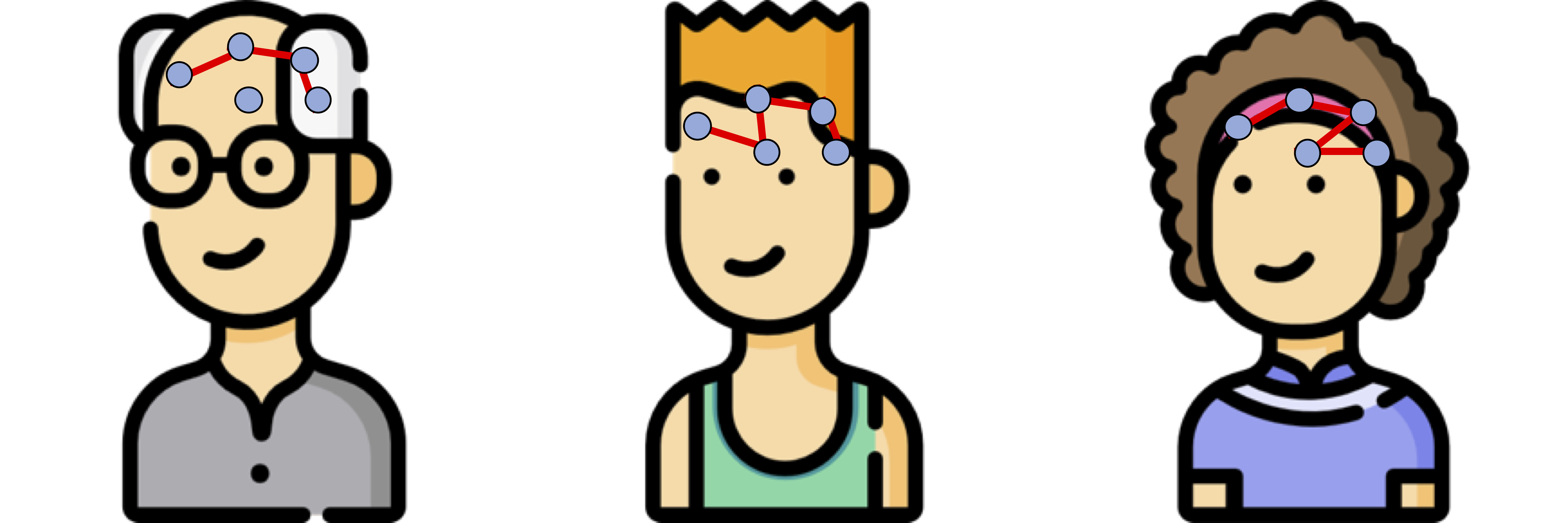Brain network connections vary by covariates (e.g. age/sex)

Goal: model how covariates change network connections

$$\textrm{function}(\textbf{graph}) = \textbf{age}\times \beta_1 + \textbf{sex}\times \beta_2 + \cdots$$

## Resting-state fMRI Networks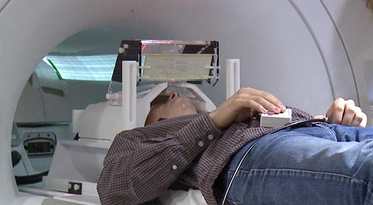• fMRI measures brain activities over time
• Resting-state: "do nothing" during scanning

• Brain networks constructed using cov/cor matrices of time series## Mathematical Problem

• Given $n$ (semi-)positive matrix outcomes, $\Sigma_i\in \real^{p\times p}$
• Given $n$ corresponding vector covariates, $x_i \in \real^{q}$
• Find function $g(\Sigma_i) = x_i \beta$, $i=1,\dotsc, n$
• In essense, regress positive matrices on vectors

## Some Related Problems

• Heterogeneous regression or weighted LS:
• Usually for scalar variance $\sigma_i$, find $g(\sigma_i) = f(x_i)$
• Goal: to improve efficiency, not to interpret $x_i \beta$
• Covariance models Anderson, 73; Pourahmadi, 99; Hoff, Niu, 12; Fox, Dunson, 15; Zou, 17
• Model $\Sigma_i = g(x_i)$, sometimes $n=i=1$
• Goal: better models for $\Sigma_i$
• Multi-group PCA Flury, 84, 88; Boik 02; Hoff 09; Franks, Hoff, 16
• No regression model, cannot handle vector $x_i$
• Goal: find common/uncommon parts of multiple $\Sigma_i$
• Tensor-on-scalar regression Li, Zhang, 17; Sun, Li, 17
• No guarantees for positive matrix outcomes

## Massive Edgewise Regressions

• Intuitive method by mostly neuroscientists
• Try $g_{j,k}(\Sigma_i) = \Sigma_{i}[j,k] = x_i \beta$
• Repeat for all $(j,k) \in \{1,\dotsc, p\}^2$ pairs
• Essentially $O(p^2)$ regressions for each connection
• Limitations: multiple testing $O(p^2)$, failure to accout for dependencies between regressions

## $\mbox{PCA}(\Sigma_i) = x_i \beta$

• Essentially, we aim to turn unsupervised PCA to a supervised PCA
• Ours differs from existing PCA methods:
• Supervised PCA Bair et al, 06 models scalar-on-vector

## Other Extensions

• Our regression framework for covariance matrix outcomes is flexbile
• The framework paper published in Biostatistics 2020
• Easily extended to voxel-level covariance matrices Brain and Behavior, Accepted
• Many other extensions under review or preparation: high dimensional, mixed effects, ...

# Model and Method

## Model

• Find principal direction (PD) $\gamma \in \real^p$, such that: $$\log({\gamma}^\top\Sigma_{i}{\gamma})=\beta_{0}+x_{i}^\top{\beta}_{1}, \quad i =1,\dotsc, n$$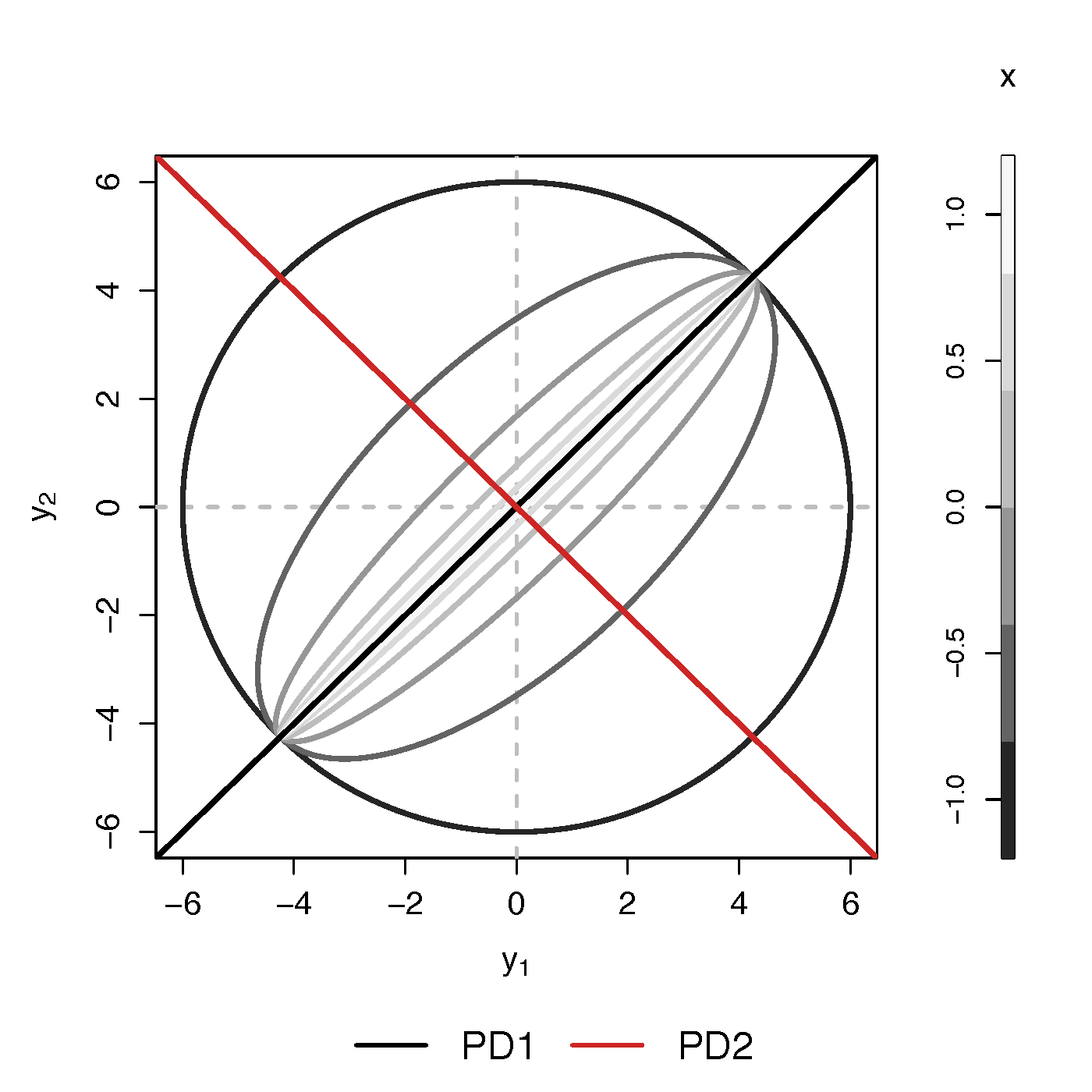Example (p=2): PD1 largest variation but not related to $x$

PCA selects PD1, Ours selects PD2

• Scalability: potentially for $p \sim 10^6$ or larger
• Interpretation: covariate assisted PCA
• Turn unsupervised PCA into supervised
• Sensitivity: target those covariate-related variations
• Covariate assisted SVD?
• Applicability: other big data problems besides fMRI

## Method

• MLE with constraints: $$\scriptsize \begin{eqnarray}\label{eq:obj_func} \underset{\boldsymbol{\beta},\boldsymbol{\gamma}}{\text{minimize}} && \ell(\boldsymbol{\beta},\boldsymbol{\gamma}) := \frac{1}{2}\sum_{i=1}^{n}(x_{i}^\top\boldsymbol{\beta}) \cdot T_{i} +\frac{1}{2}\sum_{i=1}^{n}\boldsymbol{\gamma}^\top \Sigma_{i}\boldsymbol{\gamma} \cdot \exp(-x_{i}^\top\boldsymbol{\beta}) , \nonumber \\ \text{such that} && \boldsymbol{\gamma}^\top H \boldsymbol{\gamma}=1 \end{eqnarray}$$
• Two obvious constriants:
• C1: $H = I$
• C2: $H = n^{-1} (\Sigma_1 + \cdots + \Sigma_n)$

## Choice of $H$

Proposition: When (C1) $H=\boldsymbol{\mathrm{I}}$ in the optimization problem, for any fixed $\boldsymbol{\beta}$, the solution of $\boldsymbol{\gamma}$ is the eigenvector corresponding to the minimum eigenvalue of matrix $$\sum_{i=1}^{n}\frac{\Sigma_{i}}{\exp(x_{i}^\top\boldsymbol{\beta})}$$

Will focus on the constraint (C2)

## Algoirthm

• Iteratively update $\beta$ and then $\gamma$
• Extension to multiple $\gamma$:
• After finding $\gamma^{(1)}$, we will update $\Sigma_i$ by removing its effect
• Search for the next PD $\gamma^{(k)}$, $k=2, \dotsc$
• Impose the orthogonal constraints such that $\gamma^{k}$ is orthogonal to all $\gamma^{(t)}$ for $t\lt k$

## Theory for $\beta$

Theorem: Assume $\sum_{i=1}^{n}x_{i}x_{i}^\top/n\rightarrow Q$ as $n\rightarrow\infty$. Let $T=\min_{i}T_{i}$, $M_{n}=\sum_{i=1}^{n}T_{i}$, under the true $\boldsymbol{\gamma}$, we have \begin{equation} \sqrt{M_{n}}\left(\hat{\boldsymbol{\beta}}-\boldsymbol{\beta}\right)\overset{\mathcal{D}}{\longrightarrow}\mathcal{N}\left(\boldsymbol{\mathrm{0}},2 Q^{-1}\right),\quad \text{as } n,T\rightarrow\infty, \end{equation} where $\hat{\boldsymbol{\beta}}$ is the maximum likelihood estimator when the true $\boldsymbol{\gamma}$ is known.

## Theory for $\gamma$

Theorem: Assume $\Sigma_{i}=\Gamma\Lambda_{i}\Gamma^\top$, where $\Gamma=(\boldsymbol{\gamma}_{1},\dots,\boldsymbol{\gamma}_{p})$ is an orthogonal matrix and $\Lambda_{i}=\mathrm{diag}\{\lambda_{i1},\dots,\lambda_{ip}\}$ with $\lambda_{ik}\neq\lambda_{il}$ ($k\neq l$), for at least one $i\in\{1,\dots,n\}$. There exists $k\in\{1,\dots,p\}$ such that for $\forall~i\in\{1,\dots,n\}$, $\boldsymbol{\gamma}_{k}^\top\Sigma_{i}\boldsymbol{\gamma}_{k}=\exp(x_{i}^\top\boldsymbol{\beta})$. Let $\hat{\boldsymbol{\gamma}}$ be the maximum likelihood estimator of $\boldsymbol{\gamma}_{k}$ in Flury, 84. Then assuming that the assumptions are satisfied, $\hat{ \boldsymbol{\beta}}$ from our algorithm is $\sqrt{M_{n}}$-consistent estimator of $\boldsymbol{\beta}$.

# Simulations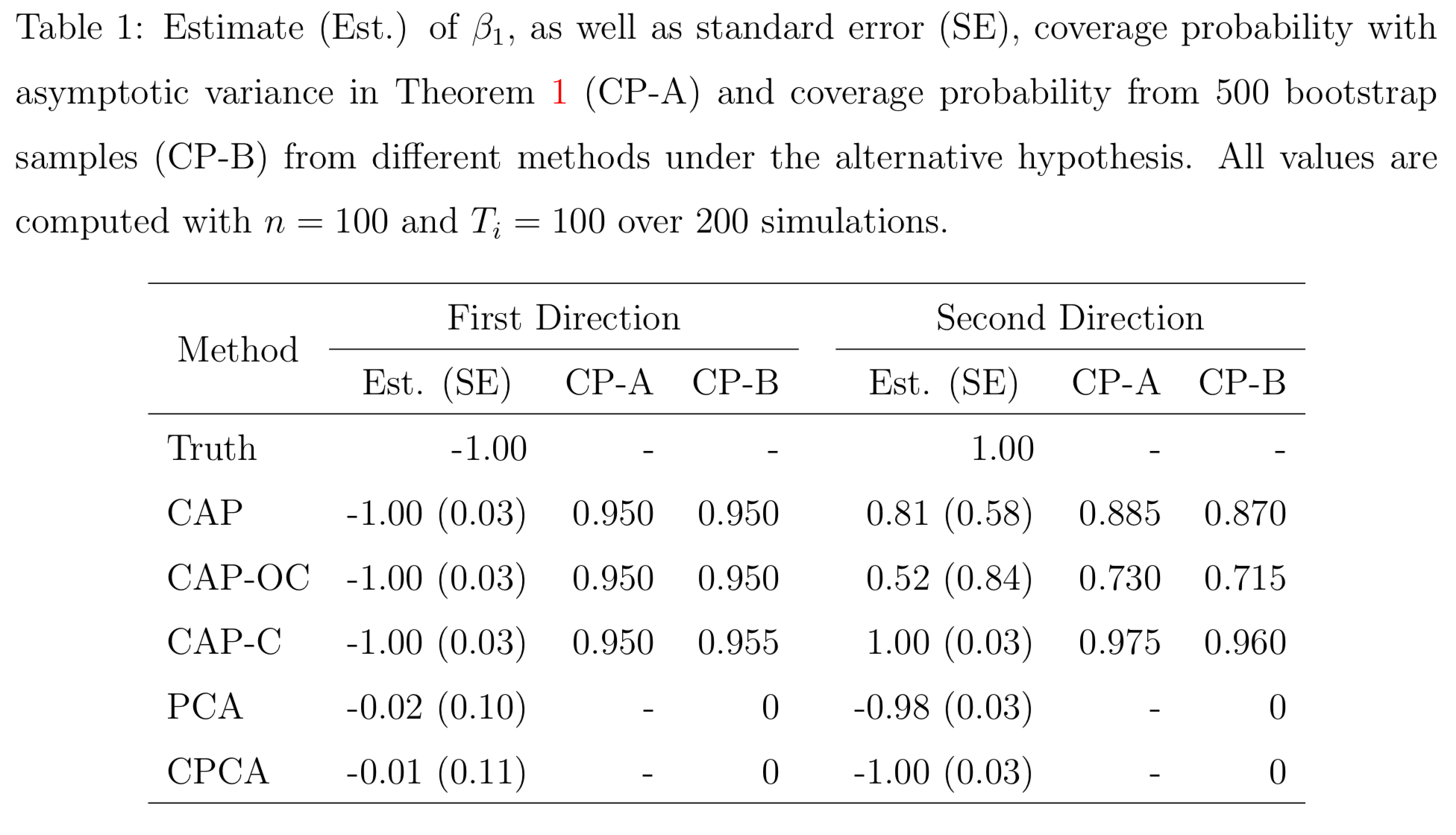PCA and common PCA do not find the first principal direction, because they don't model covariates

# Resting-state fMRI

## Regression CoefficientsAge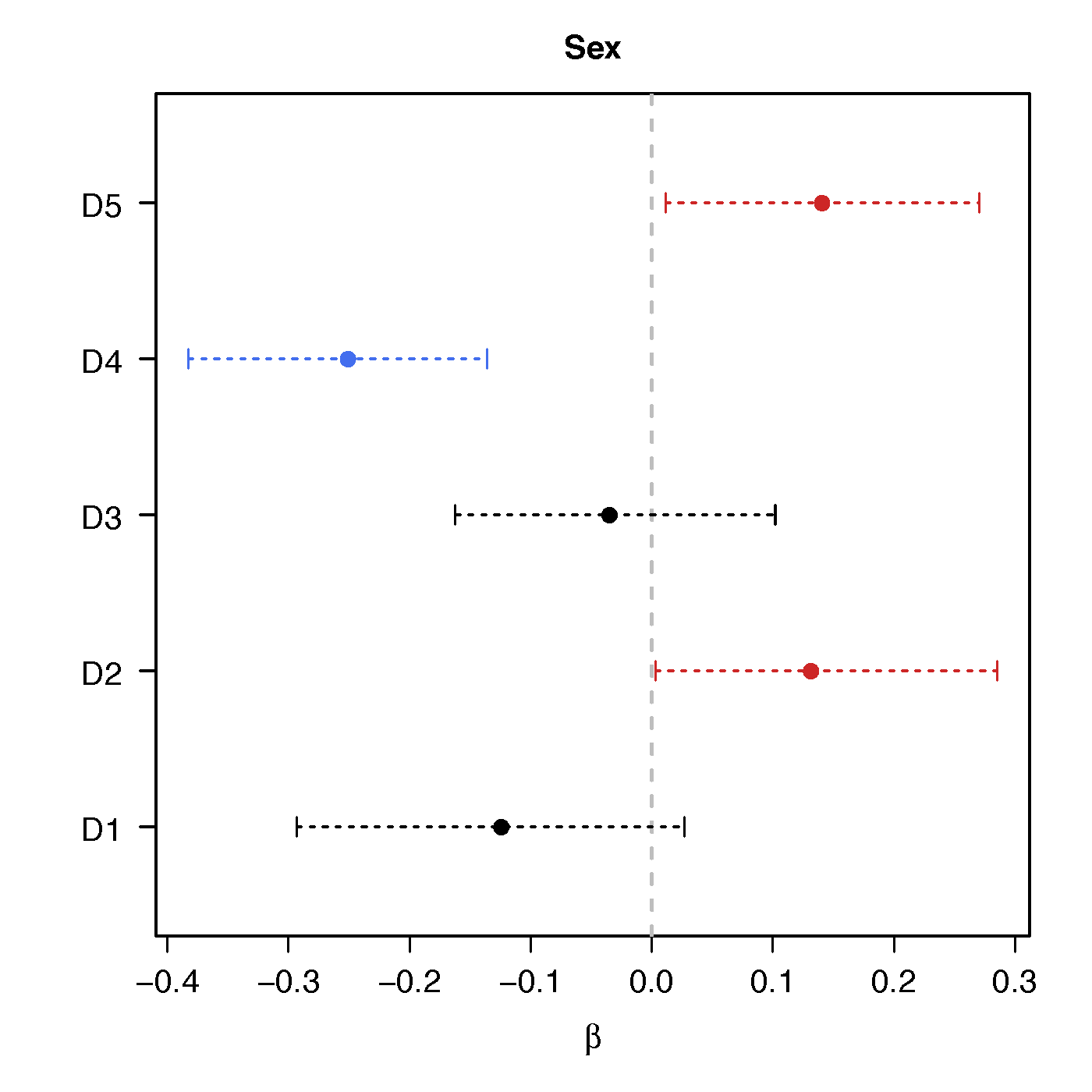Sex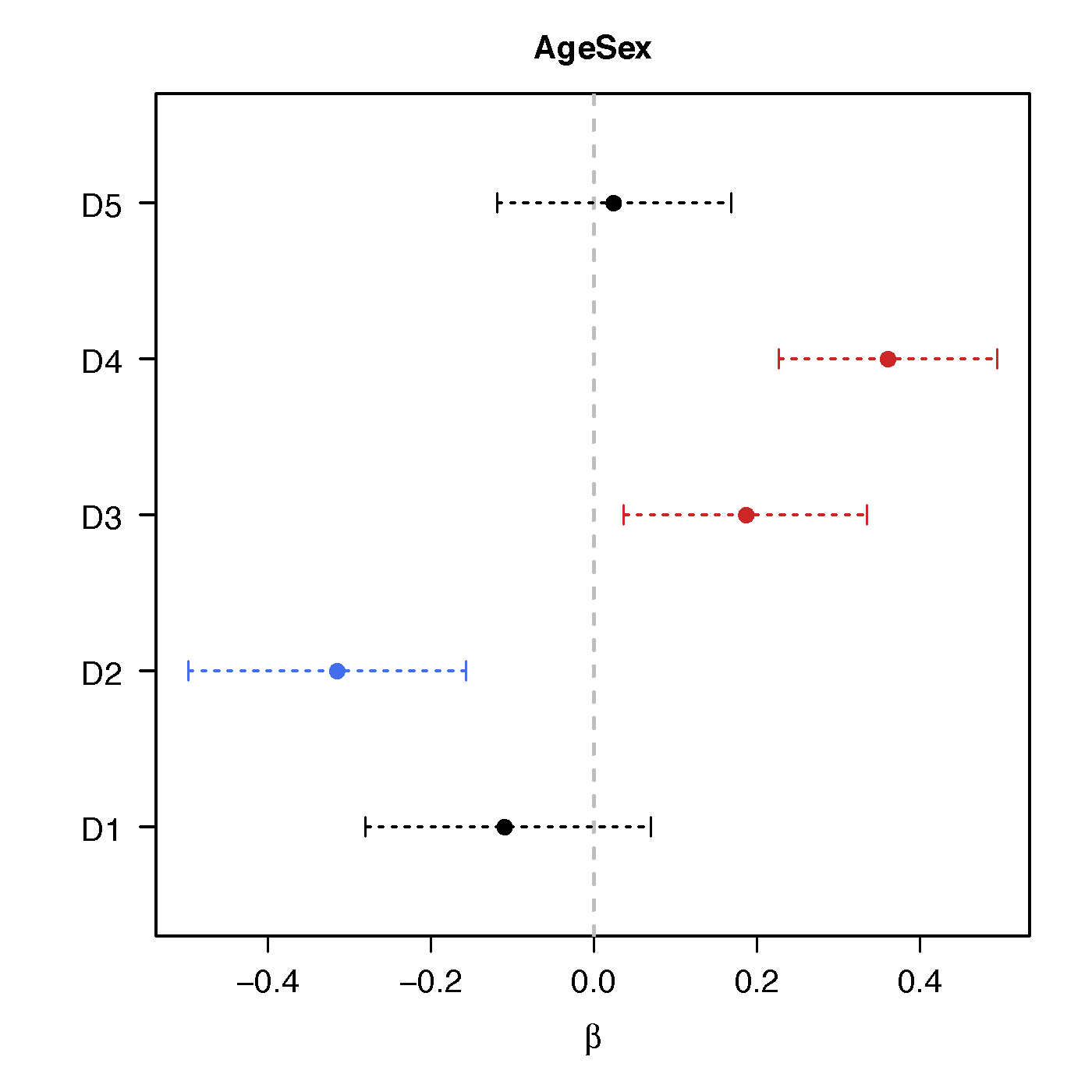Age*Sex

No statistical significant changes were found by massive edgewise regression

## Brain Map of $\gamma$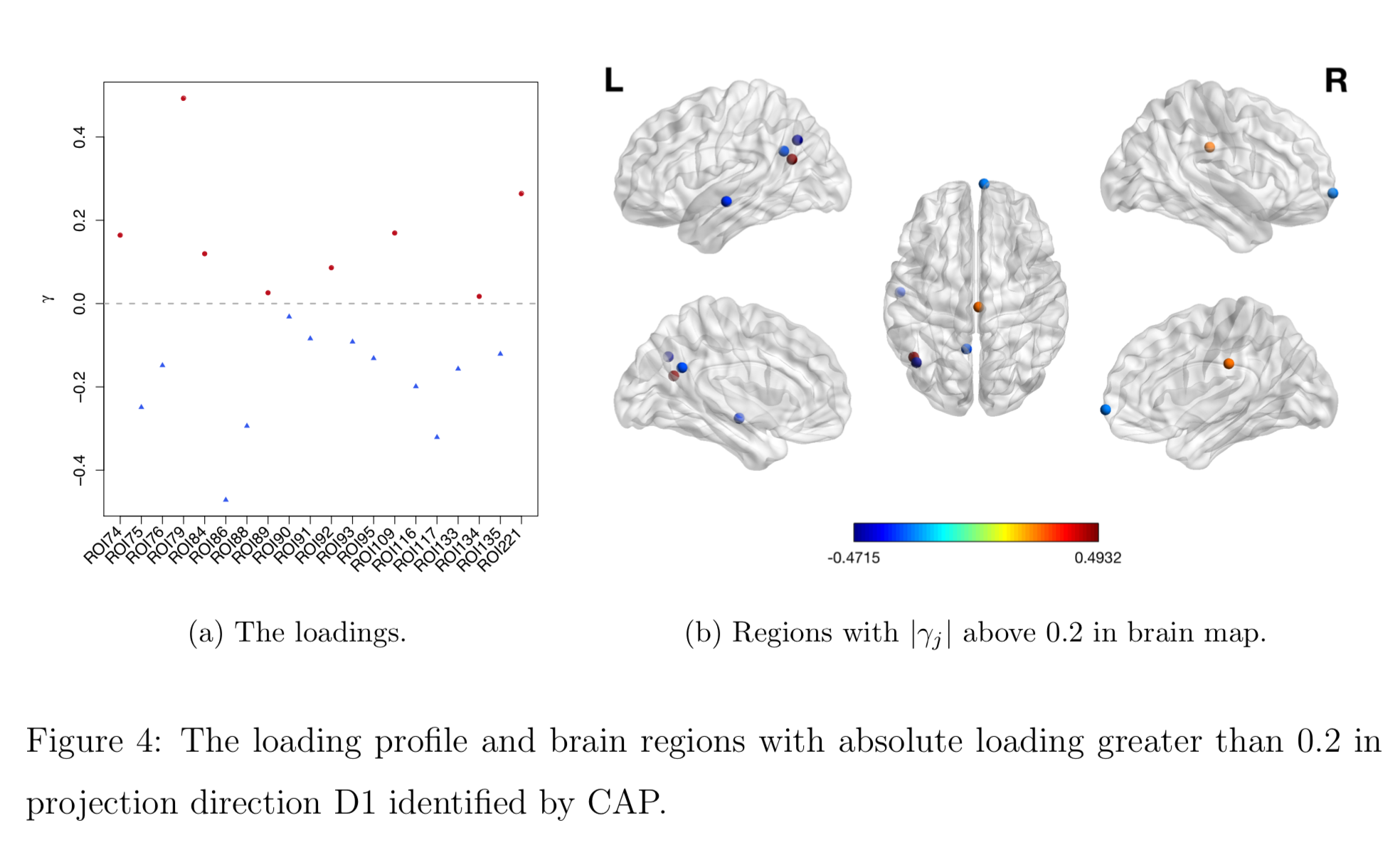## Discussion

• Regress PD matrices on vectors
• Method to identify covariate-related (supervised) directions vs (unsupervised) PCA
• Theorectical justifications
• Paper: Biostatistics (10.1093/biostatistics/kxz057)
• R pkg: cap